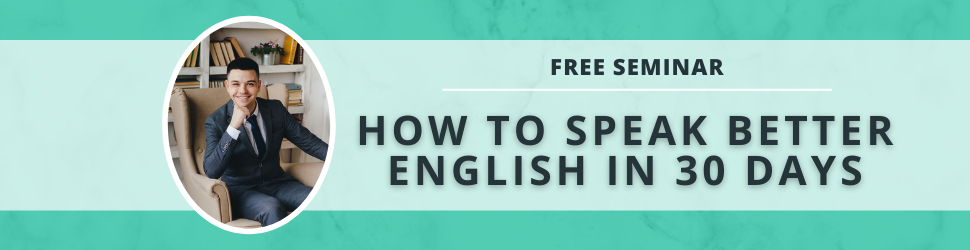# Conditional Phrases

Dec 05, 2018

Basically, conditional phrases are hypothetical phrases consisting of two clauses: an "if" clause and a "then" clause.

In this video we discuss the following conditional phrases: zero, first, second, third, and mixed.

Zero Condtional tells us about general facts or strong opinions.
Zero Conditional = If present, (then) present

Example: If it rains, you get wet.

First Conditional tells us about a possible future condition.
First Conditional = If present, (then) future

Example: If I win the lottery, I will buy a house.

Second Conditional tells us about an impossible alternative present condition.
Second Conditional = If past, (then) would + infinitive

Example: If I had a car, I would drive.

Third Conditional tells us about an impossible alternative past condition.
Third Conditional = If past perfect, (then) would + have + past participle

Example: If I had gone to the cinema, I would have watched Batman.

Mixed Conditional tells us about the relationship between an impossible alternative past condition and an impossible alternative present condition.
Mixed Conditional = If past perfect, (then) would + infinitive

Example: If Germany had won the war, we would speak German today.

I hope you benefit from this lesson! Thanks for watching!